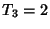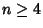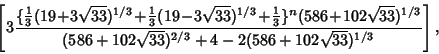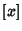## Tribonacci Number

The tribonacci numbers are a generalization of the Fibonacci Numbers defined by,,, and the Recurrence Relation(1)

for. The represent thecase of the Fibonacci n-Step Number. The first few terms are 1, 1, 2, 4, 7, 13, 24, 44, 81, 149, ... (Sloane's A000073). The ratio of adjacent terms tends to 1.83929, which is the Real Root of. The Tribonacci numbers can also be computed using the Generating Function(2)
An explicit Formula foris also given by(3)

wheredenotes the Nint function (Plouffe). The first part of a Numerator is related to the Real root of, but determination of the Denominator requires an application of the LLL Algorithm. The numbers increase asymptotically to(4)

where(5)

(Plouffe).

Plouffe, S. Tribonacci Constant.'' http://www.lacim.uqam.ca/piDATA/tribo.txt.
Sloane, N. J. A. Sequence A000073/M1074 in An On-Line Version of the Encyclopedia of Integer Sequences.'' http://www.research.att.com/~njas/sequences/eisonline.html and Sloane, N. J. A. and Plouffe, S. The Encyclopedia of Integer Sequences. San Diego: Academic Press, 1995.